Question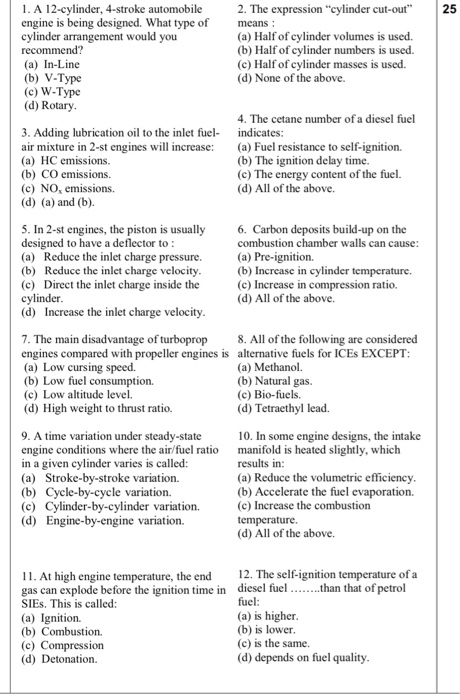1) V-type

2) Half of cylinder volume is used.

3) (a) and (b)

4) the ignition delay time

5) Increase the inlet charge velocity

6) All of the above

7) low altitude level

9) cycle by cycle variation

10) accelerate the fuel evaporation

11) detonation

12) is lower

Explanation :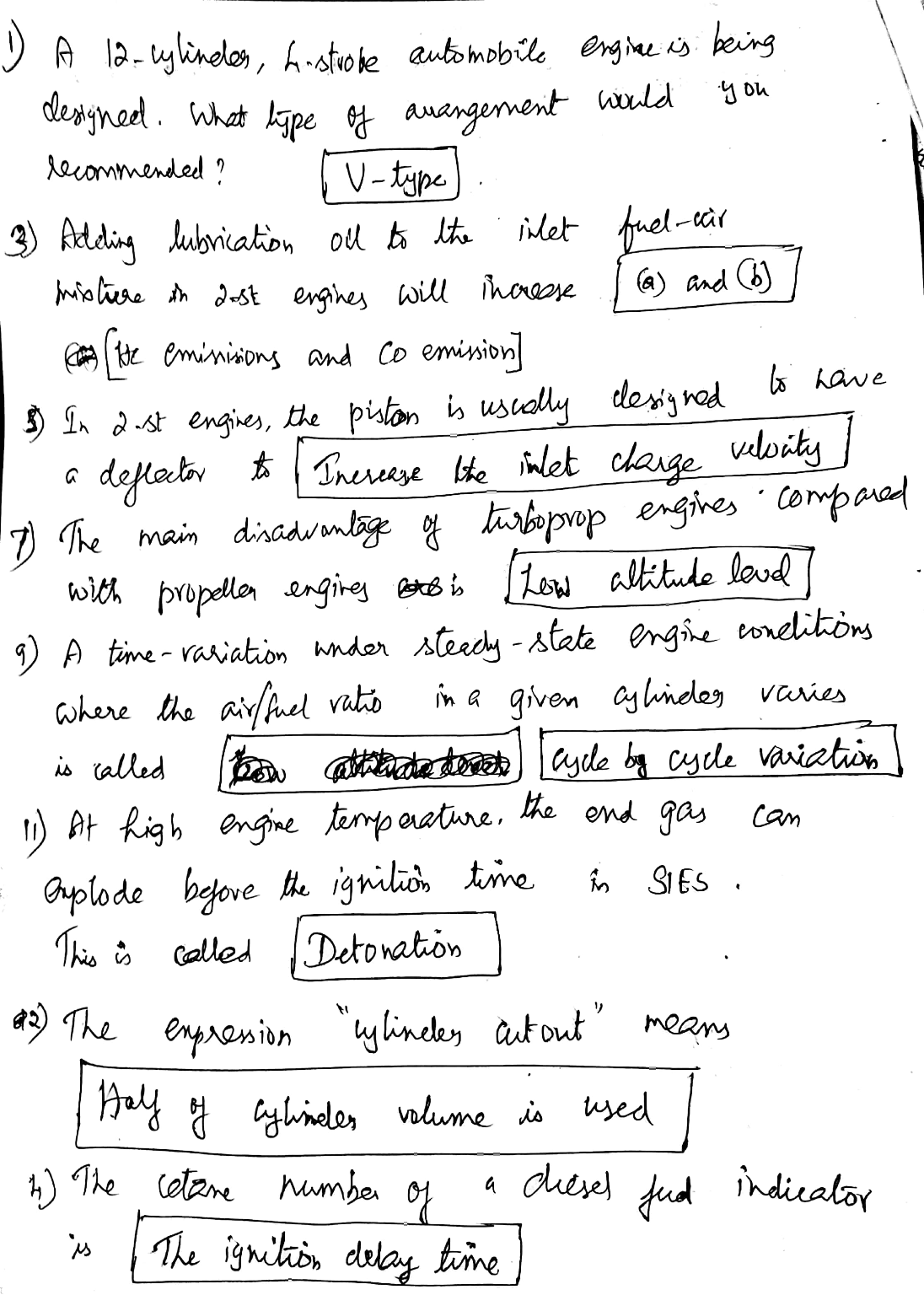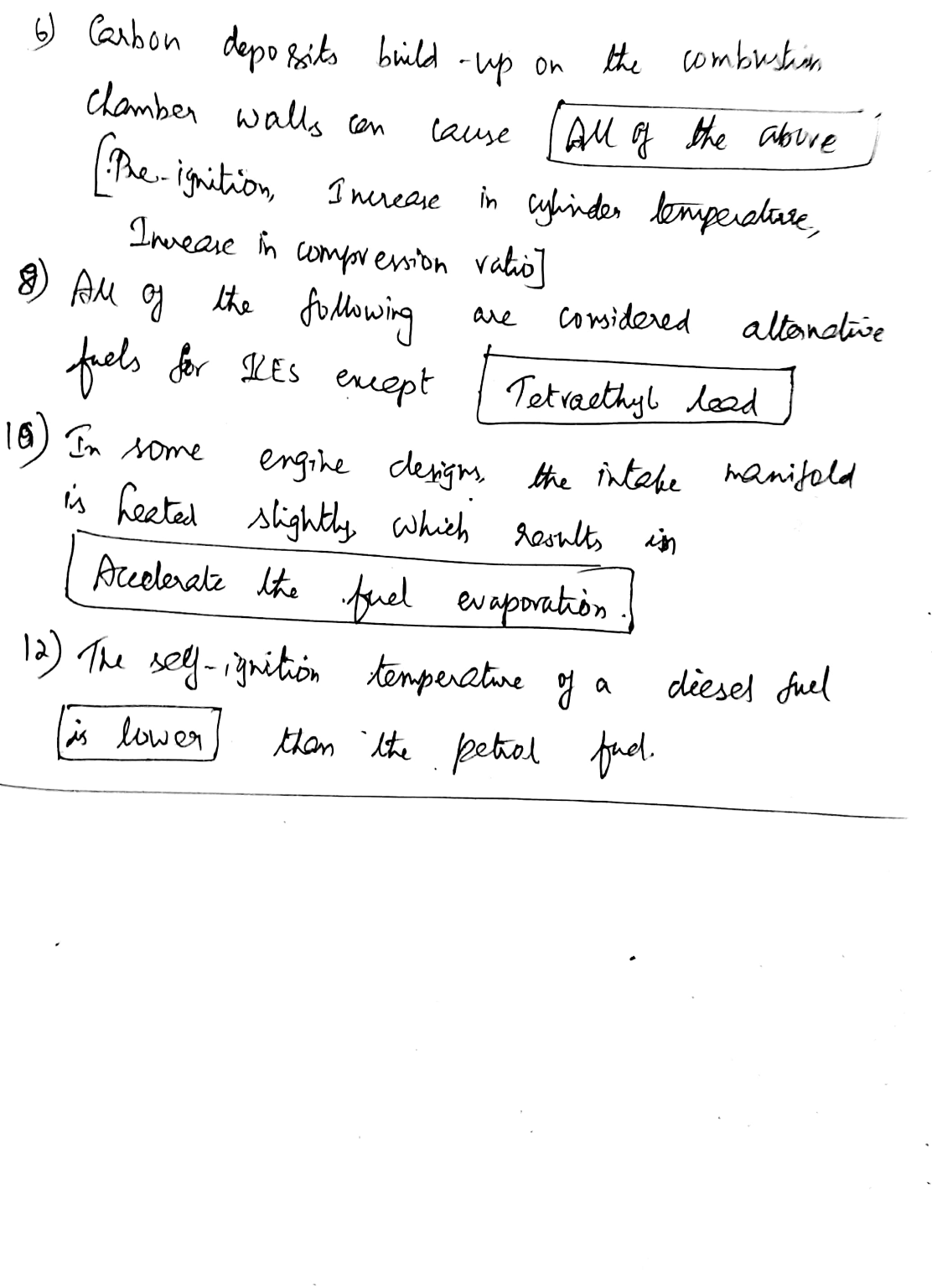#### Earn Coins

Coins can be redeemed for fabulous gifts.

Similar Homework Help Questions
• ### Which of the following is NOT included in the four-stroke cycle of a diesel engine? Choose...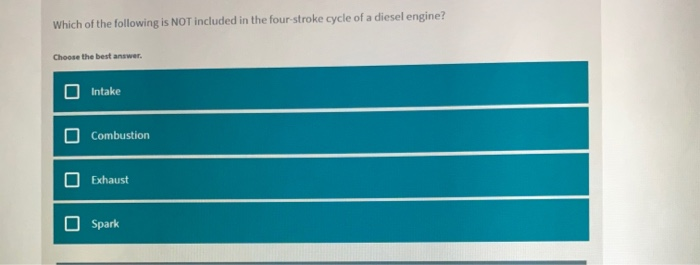Which of the following is NOT included in the four-stroke cycle of a diesel engine? Choose the best answer. Intake Combustion Exhaust Spark Regarding gas engine combustion, which of the following statements is FALSE? Choose the best answer. Gas engines have a lower compression ratio than diesel engines Gas engines are basically diesel engines with different injectors Gas engines use an ignition module and spark plug Natural gas requires more heat than diesel fuel to ignite

• ### . A 4 stroke 4 cylinder spark ignition engine runs on a stoichiometric mixture of ethanol and air...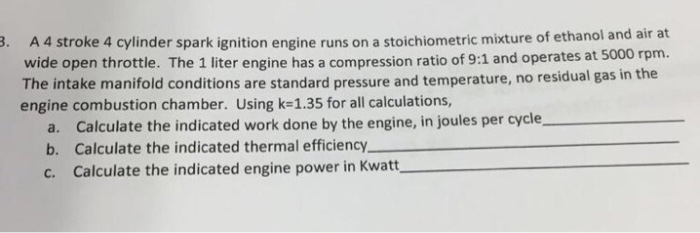. A 4 stroke 4 cylinder spark ignition engine runs on a stoichiometric mixture of ethanol and air at wide open throttle. The 1 liter engine has a compression ratio of 9:1 and operates at 5000 rpm. The intake manifold conditions are standard pressure and temperature, no residual gas in engine combustion chamber. Using k-1.35 for all calculations the a. Calculate the indicated work done by the engine, in joules per cycle b. Calculate the indicated thermal efficiency c. Calculate...

• ### Problem 6. Compute the brake mean effective pressure of a four-cylinder, two-stroke engine, 100 mm bore...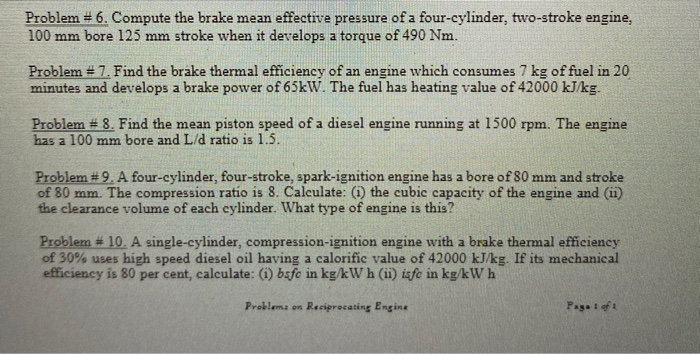Problem 6. Compute the brake mean effective pressure of a four-cylinder, two-stroke engine, 100 mm bore 125 mm stroke when it develops a torque of 490 Nm. Problem = 7. Find the brake thermal efficiency of an engine which consumes 7 kg of fuel in 20 minutes and develops a brake power of 65kW. The fuel has heating value of 42000 kJ/kg. Problem # 8. Find the mean piston speed of a diesel engine running at 1500 rpm. The engine...

• ### 1. (30P) A twenty-cylinder MTU 4 STROKE 20V 4000 M93 marine diesel engine is designed to...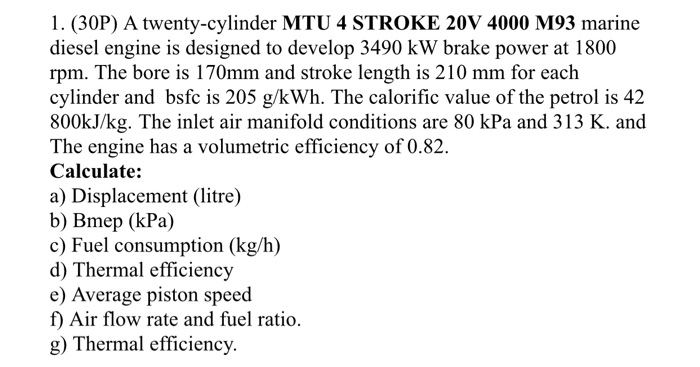1. (30P) A twenty-cylinder MTU 4 STROKE 20V 4000 M93 marine diesel engine is designed to develop 3490 kW brake power at 1800 rpm. The bore is 170mm and stroke length is 210 mm for each cylinder and bsfc is 205 g/kWh. The calorific value of the petrol is 42 800kJ/kg. The inlet air manifold conditions are 80 kPa and 313 K. and The engine has a volumetric efficiency of 0.82. Calculate: a) Displacement (litre) b) Bmep (kPa) c) Fuel...

• ### Only parts (e) and (f) is required Thanks. 2. An 8 cylinder 5.6L (total displacement) 4-stroke...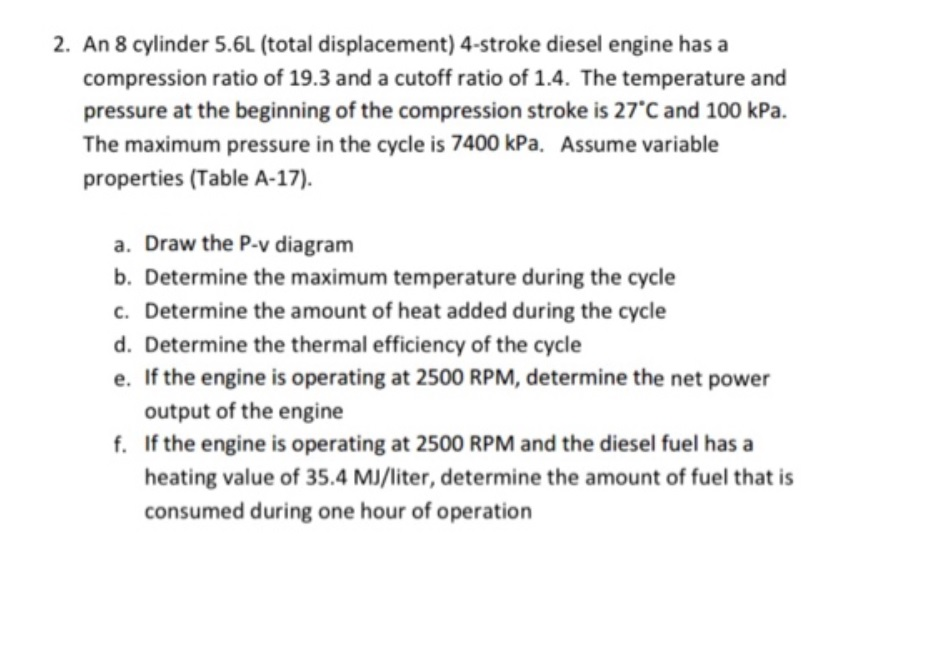Only parts (e) and (f) is required Thanks. 2. An 8 cylinder 5.6L (total displacement) 4-stroke diesel engine has a compression ratio of 19.3 and a cutoff ratio of 1.4. The temperature and pressure at the beginning of the compression stroke is 27°C and 100 kPa. The maximum pressure in the cycle is 7400 kPa. Assume variable properties (Table A-17). a. Draw the P-v diagram b. Determine the maximum temperature during the cycle c. Determine the amount of heat added...

• ### Problem 1 (65 pts): The cylinder of a Diesel engine is initially filled with air at 100 kPa and 2...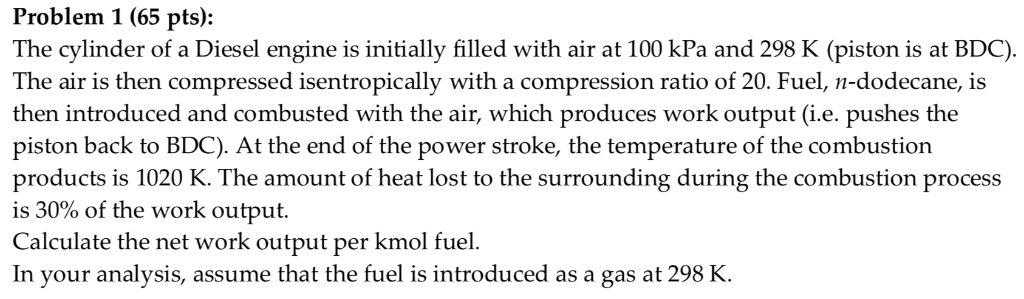Problem 1 (65 pts): The cylinder of a Diesel engine is initially filled with air at 100 kPa and 298 K (piston is at BDC). The air is then compressed isentropically with a compression ratio of 20. Fuel, n-dodecane, is then introduced and combusted with the air, which produces work output (i.e. pushes the piston back to BDC). At the end of the power stroke, the temperature of the combustion products is 1020 K. The amount of heat lost to...

• ### consider a four-cylinder, four-stroke, compression ignition engine with a compression ratio of 20 and a cutoff...

consider a four-cylinder, four-stroke, compression ignition engine with a compression ratio of 20 and a cutoff ratio of 4. A). name the thermodynamic cycle you would use to analyze this engine, b). p-v and t-s diagram for this cycle, c). determine the heat input to the engine if the temperature rise of the heat addition step is 1300 C, and d). determine the engine speed in revolutions/minute for a net power output of 40 kW is the net cycle work...

• ### INTERNAL COMBUSTION ENGINES Practice Problems ME 436 A 3 cylinder, 2.7 liter SI engine operates on...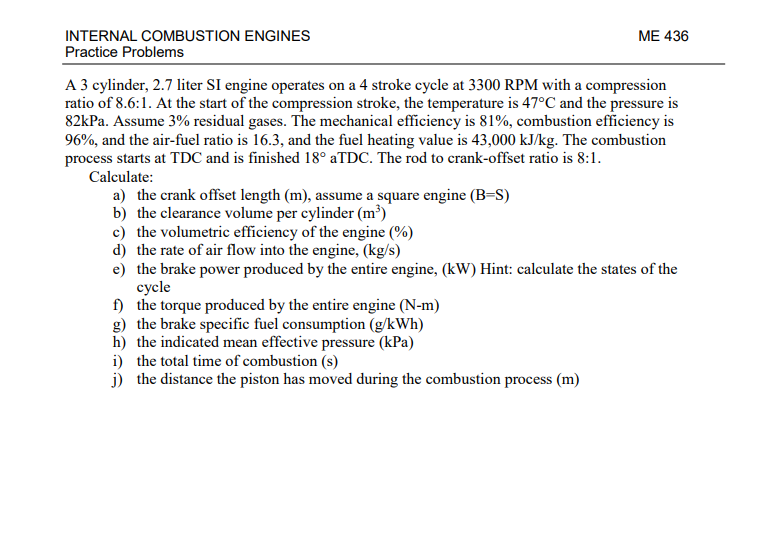INTERNAL COMBUSTION ENGINES Practice Problems ME 436 A 3 cylinder, 2.7 liter SI engine operates on a 4 stroke cycle at 3300 RPM with a compression ratio of 8.6:1. At the start of the compression stroke, the temperature is 47°C and the pressure is 82kPa. Assume 3% residual gases. The mechanical efficiency is 81%, combustion efficiency is 96%, and the air-fuel ratio is 16.3, and the fuel heating value is 43,000 kJ/kg. The combustion process starts at TDC and is...

• ### A four stroke cycle Sl engine has a cylinder condition at the start of the compression...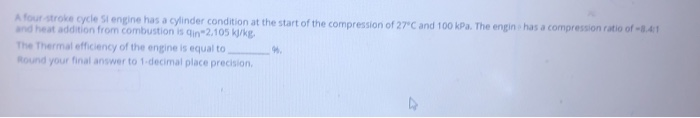A four stroke cycle Sl engine has a cylinder condition at the start of the compression of 27°C and 100 kPa. The engin has a compression ratio of -3.41 And heat addition from combustion is in "2.105 kJ/kg. The Thermal efficiency of the engine is equal to Round your final answer to 1.decimal place precision A four-stroke cycle Sl engine has a cylinder condition at the start of the compression of 27°C and 100 kPa. The engine has a compression...

• ### A four-stroke 2.0 Litre compression ignition engine operates on a Diesel cycle with a compression ratio...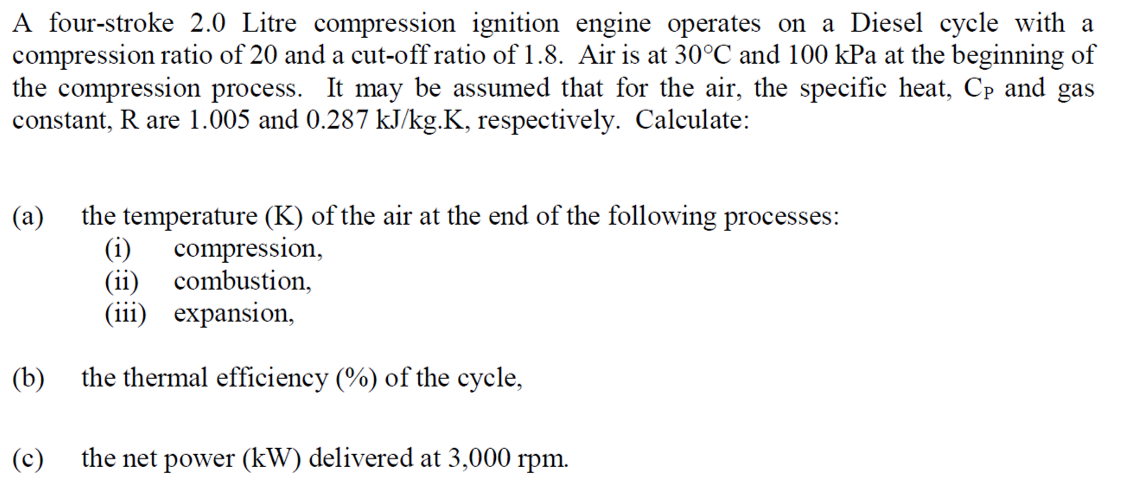A four-stroke 2.0 Litre compression ignition engine operates on a Diesel cycle with a compression ratio of 20 and a cut-off ratio of 1.8. Air is at 30°C and 100 kPa at the beginning of the compression process. It may be assumed that for the air, the specific heat, Cp and gas constant, R are 1.005 and 0.287 kJ/kg.K, respectively. Calculate: the temperature (K) of the air at the end of the following processes: (i) (a) compression (ii) combustion, (ii...